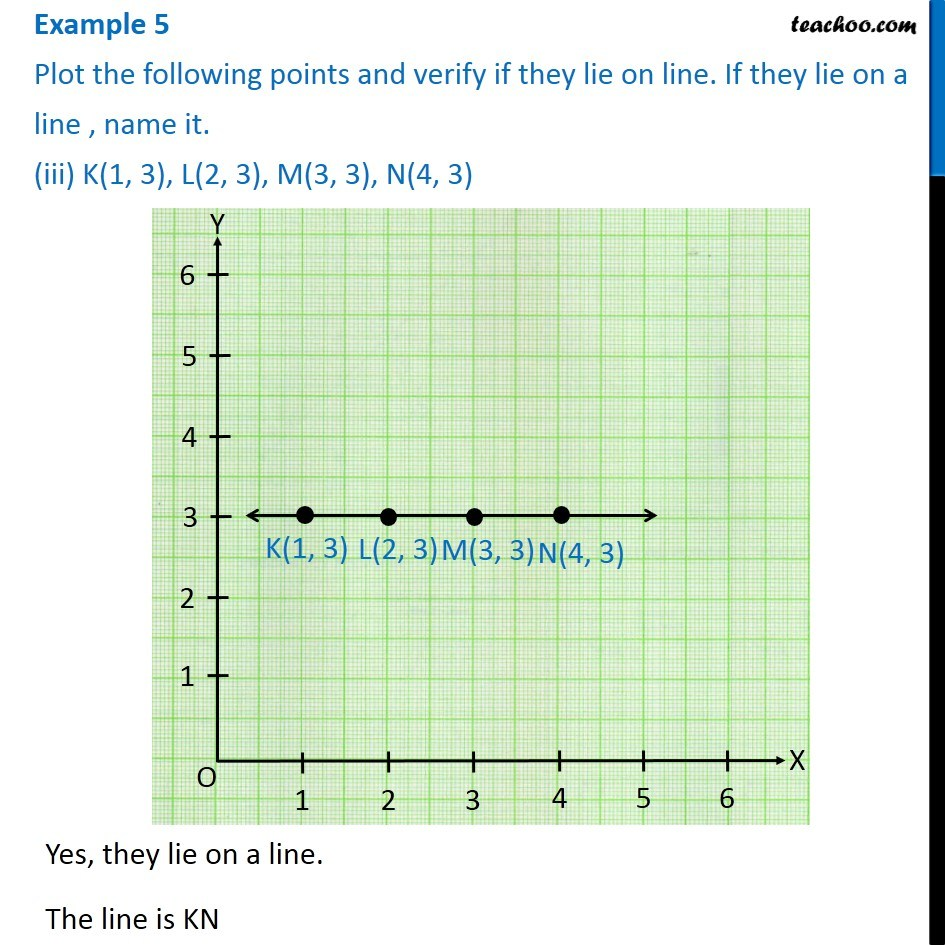1. Chapter 15 Class 8 Introduction to Graphs
2. Serial order wise
3. Examples

Transcript

Example 5 Plot the following points and verify if they lie on line. If they lie on a line , name it. (iii) K(1, 3), L(2, 3), M(3, 3), N(4, 3) Yes, they lie on a line. The line is KN